Home Practice
For learners and parents For teachers and schools
Textbooks
Full catalogue
Pricing SupportLog in

We think you are located in United States. Is this correct?

# 9.3 pH

## 9.3 pH (ESCPB)

### The pH scale (ESCPC)

The concentration of specific ions in solution determines whether the solution is acidic or basic. Acids and bases can be described as substances that either increase or decrease the concentration of hydrogen ($$\text{H}^{+}$$) or hydronium ($$\text{H}_{3}\text{O}^{+}$$) ions in a solution. An acid increases the hydrogen ion concentration in a solution, while a base decreases the hydrogen ion concentration.

pH is used to measure the concentration of $$\text{H}^{+}$$ ions ($$[\text{H}^{+}]$$) and therefore, whether a substance is acidic or basic (alkaline). Solutions with a pH of less than seven are acidic, while those with a pH greater than seven are basic (alkaline). The pH scale ranges from $$\text{0}$$ to $$\text{14}$$ and a pH of $$\text{7}$$ is considered neutral.

The universal indicator changes colour from $$\color{red}{\textbf{red}}$$ in $$\color{red}{\textbf{strongly acidic}}$$ solutions through to $$\color{purple}{\textbf{purple}}$$ in $$\color{purple}{\textbf{strongly basic}}$$ solutions.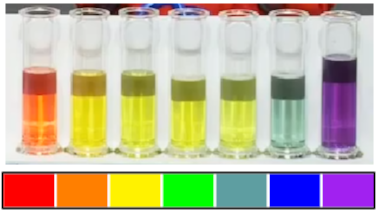The term pH was first used by in 1909 by Søren Peter Lauritz Sørensen (a Danish biochemist). The p stood for potenz and the H for hydrogen. This translates to power of hydrogen.

pH

pH is a measure of the acidity or alkalinity of a solution.

At the beginning of the chapter we mentioned that we encounter many examples of acids and bases in our day-to-day lives. The pH of solutions of some household acids and bases are given in Table 9.6.

Fizzy cooldrinks often have very low pH (are acidic).

 Drink pH Coke $$\text{2,5}$$ Diet coke $$\text{3,3}$$ Pepsi $$\text{2,5}$$ Diet pepsi $$\text{3,0}$$ Sprite $$\text{3,2}$$ 7 Up $$\text{3,2}$$ Diet 7 Up $$\text{3,7}$$
 Molecule Found in pH Type phosphoric acid fizzy drinks $$\text{2,15}$$ acid tartaric acid wine $$\text{2,95}$$ acid citric acid lemon juice $$\text{3,14}$$ acid acetic acid vinegar $$\text{4,76}$$ acid carbonic acid fizzy drinks $$\text{6,37}$$ acid ammonia cleaning products $$\text{11,5}$$ base ammonium hydroxide cleaning products $$\text{11,63}$$ base sodium hydroxide caustic soda $$\text{13}$$ base

Table 9.6: The pH of solutions of acids and bases as found in common household items.

### pH calculations (ESCPD)

The pH scale is a log scale. Remember from mathematics that a difference of one on a base $$\text{10}$$ log scale (the one on your calculator) is equivalent to a multiplication by 10. That is:

1 = log(10)

2 = log(100)

3 = log($$\text{1 000}$$)

4 = log($$\text{10 000}$$)

So a change from a pH of 2 to a pH of 6 represents a very large change in the $$\text{H}^{+}$$ concentration.

pH can be calculated using the following equation:

pH = -log[$$\text{H}^{+}$$]

[$$\text{H}^{+}$$] and [$$\text{H}_{3}\text{O}^{+}$$] can be substituted for one another:

pH = -log[$$\text{H}_{3}\text{O}^{+}$$]

The brackets in the above equation are used to show concentration in $$\text{mol·dm^{-3}}$$.

## Worked example 11: pH calculations

Calculate the pH of a solution where the concentration of hydrogen ions is

$$\text{1} \times \text{10}^{-\text{7}}$$ $$\text{mol·dm^{-3}}$$.

### Determine the concentration of hydrogen ions

In this example, the concentration has been given: $$\text{1} \times \text{10}^{-\text{7}}$$ $$\text{mol·dm^{-3}}$$

### Substitute this value into the pH equation and calculate the pH value

pH = -log[$$\text{H}^{+}$$]

= -log($$\text{1} \times \text{10}^{-\text{7}}$$)

= 7

Important: It may be useful to know that for calculations involving the pH scale, the following equations can also be used:

[$$\text{H}^{+}$$][$$\text{OH}^{-}$$] = $$\text{1} \times \text{10}^{-\text{14}}$$

[$$\text{H}_{3}\text{O}^{+}$$][$$\text{OH}^{-}$$] = $$\text{1} \times \text{10}^{-\text{14}}$$

pH = 14 - p[$$\text{OH}^{-}$$]

pH = 14 - (-log[$$\text{OH}^{-}$$])

## Worked example 12: pH calculations

In a $$\text{162}$$ $$\text{cm^{3}}$$ solution of ethanoic acid, the following equilibrium is established:

$$\text{CH}_{3}\text{COOH}(\text{aq}) + \text{H}_{2}\text{O}(\text{l})$$ $$\rightleftharpoons$$ $$\text{CH}_{3}\text{COO}^{-}(\text{aq}) + \text{H}_{3}\text{O}^{+}(\text{aq})$$

The number of moles of $$\text{CH}_{3}\text{COO}^{-}$$ is found to be $$\text{0,001}$$ $$\text{mol}$$. Calculate the pH of the solution.

### Determine the number of moles of hydronium ions in the solution

According to the balanced equation for this reaction, the mole ratio of $$\text{CH}_{3}\text{COO}^{-}$$ ions to $$\text{H}_{3}\text{O}^{+}$$ ions is $$\text{1}$$:$$\text{1}$$, therefore the number of moles of these two ions in the solution will be the same.

So, $$n(\text{H}_{3}\text{O}^{+}) = \text{0,001} \text{ mol}$$.

### Determine the concentration of hydronium ions in the solution

$$c\text{ (mol·dm}^{-3}{\text{)}} = \dfrac{n\text{ (mol)}}{ V\text{ (dm}^{3}{\text{)}}}$$

$$V = \text{162}$$ $$\text{cm^{3}}$$ $$\times \dfrac{\text{0,001} {\text{ dm}}^{3}}{\text{1} {\text{ cm}}^{3}}$$ = $$\text{0,162}$$ $$\text{dm^{3}}$$

[$$\text{H}_{3}\text{O}^{+}$$] $$= \dfrac{\text{0,001} {\text{ mol}}}{\text{0,162} {\text{ dm}}^{3}} =$$ $$\text{0,0062}$$ $$\text{mol·dm^{-3}}$$

### Substitute this value into the pH equation and calculate the pH value

pH = -log[$$\text{H}^{+}$$] = -log[$$\text{H}_{3}\text{O}^{+}$$]

$$= -$$log($$\text{0,0062}$$)

$$= \text{2,21}$$

temp text

Understanding pH is very important. In living organisms, it is necessary to maintain a constant pH in the optimal range for that organism, so that chemical reactions can occur.

 pH $$\text{1}$$ $$\text{6}$$ $$\text{7}$$ $$\text{8}$$ $$\text{13}$$ $$[\text{H}^{+}]$$ $$\text{1} \times \text{10}^{-\text{1}}$$ $$\text{1} \times \text{10}^{-\text{6}}$$ $$\text{1} \times \text{10}^{-\text{7}}$$ $$\text{1} \times \text{10}^{-\text{8}}$$ $$\text{1} \times \text{10}^{-\text{13}}$$ $$[\text{OH}^{-}]$$ $$\text{1} \times \text{10}^{-\text{13}}$$ $$\text{1} \times \text{10}^{-\text{8}}$$ $$\text{1} \times \text{10}^{-\text{7}}$$ $$\text{1} \times \text{10}^{-\text{6}}$$ $$\text{1} \times \text{10}^{-\text{1}}$$ Solution $$\color{red}{\textbf{strongly acidic}}$$ $$\color{red}{\text{weakly acidic}}$$ neutral $$\color{blue}{\text{weakly basic}}$$ $$\color{blue}{\textbf{strongly basic}}$$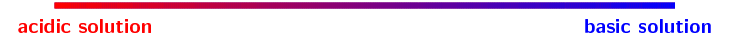Table 9.7: The concentration of $$[\text{H}^{+}]$$ and $$[\text{OH}^{-}]$$ ions in solutions with different pH.

A build up of acid in the human body can be very dangerous. Lactic acidosis is a condition caused by the buildup of lactic acid in the body. It leads to acidification of the blood (acidosis) and can make a person very ill. Some of the symptoms of lactic acidosis are deep and rapid breathing, vomiting, and abdominal pain. In the fight against HIV, lactic acidosis is a problem. One of the antiretrovirals (ARV's) that is used in anti-HIV treatment is Stavudine (also known as Zerit or d4T). One of the side effects of Stavudine is lactic acidosis, particularly in overweight women. If it is not treated quickly, it can result in death.

Litmus paper can be used as a pH indicator. It is sold in strips. Purple litmus paper will become red in acidic conditions and blue in basic conditions. Blue litmus paper is used to detect acidic conditions, while red litmus paper is used to detect basic conditions.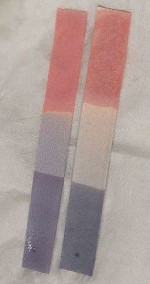In agriculture, it is important for farmers to know the pH of their soils so that they are able to plant the right kinds of crops. The pH of soils can vary depending on a number of factors, such as rainwater, the kinds of rocks and materials from which the soil was formed and also human influences such as pollution and fertilisers. The pH of rain water can also vary, and this too has an effect on agriculture, buildings, water courses, animals and plants. Rainwater is naturally acidic because carbon dioxide in the atmosphere combines with water to form carbonic acid. Unpolluted rainwater has a pH of approximately $$\text{5,6}$$. However, human activities can alter the acidity of rain and this can cause serious problems such as acid rain.

## Calculating pH

Textbook Exercise 9.5

Calculate the pH of each of the following solutions:

(Tip: $$[\text{H}_{3}\text{O}^{+}][\text{OH}^{-}]$$ = $$\text{1} \times \text{10}^{-\text{14}}$$ can be used to determine $$[\text{H}_{3}\text{O}^{+}]$$)

A $$\text{KOH}$$ solution with a $$\text{0,2}$$ $$\text{mol·dm^{-3}}$$ concentration of $$\text{OH}^{-}$$.

Remember that $$[\text{OH}^{-}][\text{H}_{3}\text{O}^{+}]$$ = $$\text{1} \times \text{10}^{-\text{14}}$$.

Therefore $$[\text{H}_{3}\text{O}^{+}]$$ = $$\dfrac{\text{1} \times \text{10}^{-\text{14}}}{{\text{[OH}}^{-}{\text{]}}}$$

$$[\text{H}_{3}\text{O}^{+}]$$ = $$\dfrac{\text{1} \times \text{10}^{-\text{14}}}{\text{0,2}}$$

$$[\text{H}_{3}\text{O}^{+}]$$ = $$\text{5} \times \text{10}^{-\text{14}}$$ $$\text{mol·dm^{-3}}$$

pH $$= -$$log[$$\text{H}_{3}\text{O}^{+}$$]

pH $$= -$$log($$\text{5} \times \text{10}^{-\text{14}}$$)

pH $$= \text{13,3}$$

OR

p[$$\text{OH}^{-}$$] $$= -$$log[$$\text{0,2}$$ $$\text{mol·dm^{-3}}$$]

p[$$\text{OH}^{-}$$] $$= \text{0,699}$$

pH $$= 14 -$$ p[$$\text{OH}^{-}$$]

pH $$= 14 - \text{0,699}$$

pH $$= \text{13,3}$$

An aqueous solution with a $$\text{1,83} \times \text{10}^{-\text{7}}$$ $$\text{mol·dm^{-3}}$$ concentration of $$\text{HCl}$$ molecules at equilibrium ($$\text{K}_{\text{a}}$$ = $$\text{1,3} \times \text{10}^{\text{6}}$$)

To determine the concentration of $$\text{H}_{3}\text{O}^{+}$$ ions in solution we must first write the expression for $$\text{K}_{\text{a}}$$, and to do that we need the balanced equation:

$$\text{HCl}(\text{aq}) + \text{H}_{2}\text{O}(\text{l})$$ $$\to$$ $$\text{H}_{3}\text{O}^{+}(\text{aq}) + \text{Cl}^{-}(\text{aq})$$

$$\text{K}_{\text{a}} = \dfrac{\text{[H}_{3}{\text{O}}^{+}{\text{][Cl}}^{-}{\text{]}}}{\text{[HCl]}}$$

$$[\text{H}_{3}\text{O}^{+}]$$ = $$\dfrac{\text{K}_{\text{a}} \times {\text{[HCl]}}}{\text{[Cl}^{-}{\text{]}}}$$

The concentration of $$\text{H}_{3}\text{O}^{+}$$ will be the same as the concentration of $$\text{Cl}^{-}$$, therefore we can say:

$$[\text{H}_{3}\text{O}^{+}]^{2}$$ = $$\text{K}_{\text{a}}$$ x $$[\text{HCl}]$$ = $$\text{1,3} \times \text{10}^{\text{6}}$$ x $$\text{1,83} \times \text{10}^{-\text{7}}$$ = $$\text{0,24}$$

$$[\text{H}_{3}\text{O}^{+}]$$ = $$\text{0,49}$$ $$\text{mol·dm^{-3}}$$

pH $$= -$$log[$$\text{H}_{3}\text{O}^{+}$$]

pH $$= -$$log($$\text{0,49}$$)

pH $$= \text{0,31}$$

What is the concentration of $$\text{H}_{3}\text{O}^{+}$$ ions in a solution with a pH of $$\text{12}$$?

pH = -log[$$\text{H}^{+}$$] = -log[$$\text{H}_{3}\text{O}^{+}$$]

Therefore log[$$\text{H}_{3}\text{O}^{+}$$] = -pH

log[$$\text{H}_{3}\text{O}^{+}$$] = $$-\text{12}$$

[$$\text{H}_{3}\text{O}^{+}$$] = $$\text{1} \times \text{10}^{-\text{12}}$$ $$\text{mol·dm^{-3}}$$

In a typical sample of seawater the concentration the hydronium ($$\text{H}_{3}\text{O}^{+}$$) ions is $$\text{1} \times \text{10}^{-\text{8}}$$ $$\text{mol·dm^{-3}}$$, while the concentration of the hydroxide ($$\text{OH}^{-}$$) ions is $$\text{1} \times \text{10}^{-\text{6}}$$ $$\text{mol·dm^{-3}}$$.

Is the seawater acidic or basic?

The concentration of $$\text{H}_{3}\text{O}^{+}$$ is lower than that of $$\text{OH}^{-}$$. The seawater is therefore basic.

What is the pH of the seawater?

pH = -log[$$\text{H}_{3}\text{O}^{+}$$]

pH = -log($$\text{1} \times \text{10}^{-\text{8}}$$)

pH = 8

This confirms that the seawater is slightly basic

Give a possible explanation for the pH of the seawater.

Mineral salts are generally alkaline. There are lots of mineral salts dissolved in the ocean and this leads to alkaline (basic) seawater.

### $$K_{w}$$ (ESCPF)

Water ionises to a very small extent:

$$\text{H}_{2}\text{O}(\text{l}) + \text{H}_{2}\text{O}(\text{l})$$ $$\rightleftharpoons$$ $$\text{H}_{3}\text{O}^{+}(\text{aq}) + \text{OH}^{-}(\text{aq})$$

This type of reaction (the transfer of a proton between identical molecules) is known as auto-protolysis. This reaction is also known as the auto-ionisation of water and the ions formed are a conjugate acid and base pair of water: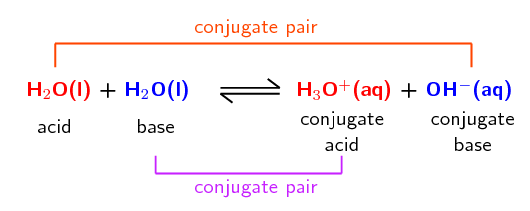Auto-protolysis and auto-ionisation of water

Auto-protolysis is the transfer of a proton between two of the same molecules. The auto-ionisation of water is one example of auto-protolysis.

$$\text{K}_{\text{w}}$$ is the equilibrium constant for this process:

$$\text{K}_{\text{w}}$$ = $$[\text{H}_{3}\text{O}^{+}][\text{OH}^{-}]$$

At $$\text{25}$$ $$\text{℃}$$: $$[\text{H}_{3}\text{O}^{+}]$$ = [$$\text{H}^{+}$$] = $$\text{1} \times \text{10}^{-\text{7}}$$, therefore:

$$\text{K}_{\text{w}}$$ = $$\text{1} \times \text{10}^{-\text{14}}$$ at $$\text{25}$$ $$\text{℃}$$

### Salt hydrolysis (ESCPG)

Does neutralisation mean that the pH of the solution is $$\text{7}$$? No. At the equivalence point of a reaction, the pH of the solution need not be $$\text{7}$$. This is because of the interaction of the salt (formed by the reaction) and water.

At the equivalence point of an acid-base neutralisation reaction there is salt and water. The ions of water interact with the salt present and form a small quantity of excess hydronium ions ($$\text{H}_{3}\text{O}^{+}$$) or hydroxide ions ($$\text{OH}^{-}$$). This leads to pH values that are not equal to $$\text{7}$$.

A simple rule for determining the likely pH of a solution is as follows:

A neutralisation reaction does not imply that the pH is neutral ($$\text{7}$$).

• A $$\color{red}{\textbf{strong }\text{acid}} + \color{blue}{\textbf{strong }\text{base}}$$ form a $$\color{darkgreen}{\textbf{neutral}}$$ salt and water $$\color{darkgreen}{\textbf{solution}}$$:

$$\to$$ pH = $$\text{7}$$.

$$\color{red}{\text{H}_{2}{\text{SO}}_{4}{\text{(aq)}}} + 2\color{blue}{\text{NaOH(s)}} \to \color{darkgreen}{\textbf{Na}_{2}{\textbf{SO}}_{4}{\textbf{(aq)}}} + 2\color{darkgreen}{\text{H}_{2}{\text{O(ℓ)}}}$$

• A $$\color{red}{\textbf{weak }\text{acid}} + \color{blue}{\textbf{strong }\text{base}}$$ form a $$\color{blue}{\textbf{weak basic}}$$ salt and water $$\color{blue}{\textbf{solution}}$$:

$$\to$$ pH = approximately $$\text{9}$$.

$$\color{red}{\text{HF(aq)}} + \color{blue}{\text{NaOH(s)}} \to \color{blue}{\textbf{NaF(aq)}} + \color{blue}{\text{H}_{2}{\text{O(ℓ)}}}$$

• A $$\color{red}{\textbf{strong }\text{acid}} + \color{blue}{\textbf{weak }\text{base}}$$ form a $$\color{red}{\textbf{weak acidic}}$$ salt $$\color{red}{\textbf{solution}}$$:

$$\to$$ pH = approximately $$\text{5}$$.

$$\color{red}{\text{H}_{2}{\text{SO}}_{4}{\text{(aq)}}} + 2\color{blue}{\text{NH}_{3}{\text{(ℓ)}}} \to \color{red}{\textbf{(NH}_{4}{\textbf{)}}_{2}{\textbf{SO}}_{4}{\textbf{(aq)}}}$$

 $$\color{red}{\textbf{acid}}$$ $$\color{blue}{\textbf{base}}$$ solution approximate pH strong strong $$\color{darkgreen}{\textbf{neutral}}$$ $$\text{7}$$ weak strong $$\color{blue}{\textbf{weak basic}}$$ $$\text{9}$$ strong weak $$\color{red}{\textbf{weak acidic}}$$ $$\text{5}$$

Table 9.8: The approximate pH of neutralisation reaction solutions based on the strength of the acid and base used.

### Indicators (ESCPH)

A titration is a process for determining, with precision, the concentration of a solution with unknown concentration. The theory behind titrations will be discussed later in this chapter. An indicator is used to show the scientist carrying out the reaction exactly when the reaction has reached completion.

This experiment looks at the change in colour of an indicator during an acid-base reaction. It is effectively a very rough titration experiment. Principles that can be applied to titrations, such as adding a small volume of acid, then swirling, can be applied here as well. It is important that the learners understand that the pH range that the indicator changes colour in is not always around $$\text{7}$$.

Learners are working with a strong acid and a strong base in this reaction. Concentrated, strong acids and bases can cause serious burns. Please remind the learners to be careful and wear the appropriate safety equipment when handling all chemicals, especially concentrated acids and bases. The safety equipment includes gloves, safety glasses, and protective clothing.

## Indicators

### Aim

To investigate the use of an indicator in an acid-base reaction.

### Apparatus and materials

• one volumetric flask, one conical flask, one pipette, a piece of white paper or a white tile

• A $$\text{1}$$ $$\text{mol·dm^{-3}}$$ solution of sodium hydroxide ($$\text{NaOH}$$), a $$\text{1}$$ $$\text{mol·dm^{-3}}$$ solution of hydrochloric ($$\text{HCl}$$), an indicator

### Method

1. Measure $$\text{20}$$ $$\text{ml}$$ of the sodium hydroxide solution into a conical flask. Add a few drops of the indicator.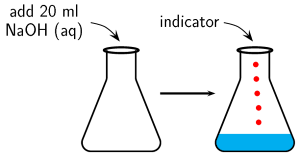2. In this experiment colour change is very important. So place the conical flask on a piece of white paper or a white tile to make any colour change easier to observe.

3. Slowly add $$\text{10}$$ $$\text{ml}$$ of hydrochloric acid. If there is a colour change, then stop. If there is no colour change add another $$\text{5}$$ $$\text{ml}$$. Continue adding $$\text{5}$$ $$\text{ml}$$ increments until you notice a colour change.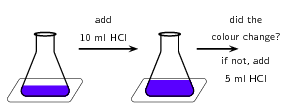### Observations and discussion

The solution changes colour after a certain amount of hydrochloric acid is added. This is because the solution now contains more acid than base and has therefore become acidic. It can be concluded that the indicator is one colour in a basic solution and a different colour in an acidic solution.

Indicators are chemical compounds that change colour depending on whether they are in an acidic or a basic solution. A titration requires an indicator that will respond to the change in pH with a sensitive and quick colour change. Typical indicators used in titrations are given in Table 9.9.

 Titration type Preferred indicator Colour of acid Colour of end-point Colour of base pH range strong acid + strong base bromothymol blue yellow green blue $$\text{6,0}$$ - $$\text{7,6}$$ weak acid + strong base phenolphthalein colourless faint pink pink $$\text{8,3}$$ - $$\text{10,0}$$ strong acid + weak base bromocresol green yellow green blue $$\text{3,8}$$ - $$\text{5,4}$$

Table 9.9: Some typical indicators for typcial titrations.

Revise Grade 11 Acids and Bases for more information on plants and foods that can be used as indicators.

Notice that the pH range for colour change of the indicator used should match the approximate pH expected for that type of titration (see Table 9.8):

 solution pH pH range neutral $$\text{7}$$ $$\text{6,0}$$ - $$\text{7,6}$$ weak basic $$\text{9}$$ $$\text{8,3}$$ - $$\text{10,0}$$ weak acidic $$\text{5}$$ $$\text{3,8}$$ - $$\text{5,4}$$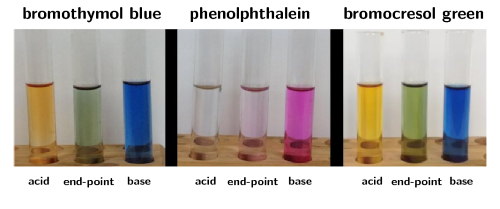Figure 9.3: Some typical indicators for typcial titrations: bromothymol blue (left), phenolphthalein (centre), and bromocresol green (right).

Indicators change colour (Figure 9.3) according to where the $$\text{H}$$ is: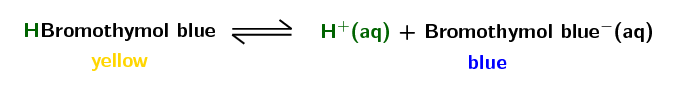So, when an acid is added to aqueous bromothymol blue there will be extra $$\text{H}^{+}$$ ions. The equilibrium will shift (remember le Chatalier's principle) to decrease the number of $$\text{H}^{+}$$ ions. That is, to the left. If sufficient acid is added, the entire solution will become acidic. This means there will be more HBromothymol blue than bromothymol blue$$^{-}$$ and the solution will become yellow.